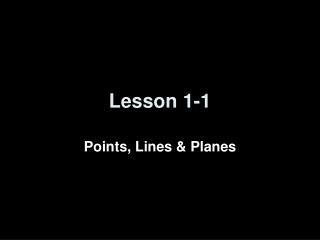DownloadDownload PresentationLesson 1-1

# Lesson 1-1

Télécharger la présentation## Lesson 1-1

- - - - - - - - - - - - - - - - - - - - - - - - - - - E N D - - - - - - - - - - - - - - - - - - - - - - - - - - -
##### Presentation Transcript

1. Lesson 1-1 Points, Lines & Planes

2. Transparency 1-1 y x k (6,4) C (0,1) (-6,-2) A B 5-Minute Check on Algebra • 6x + 45 = 18 – 3x • x2 – 45 = 4 • (3x + 4) + (4x – 7) = 11 • (4x –10) + (6x +30) = 180 • Find the slope of the line k. • Find the slope of a perpendicular line to k Standardized Test Practice: A B C D -2 1/2 2 -1/2 Click the mouse button or press the Space Bar to display the answers.

3. Transparency 1-1 y x k (6,4) C (0,1) (-6,-2) A B 5-Minute Check on Algebra • 6x + 45 = 18 – 3x • x2 – 45 = 4 • (3x + 4) + (4x – 7) = 11 • (4x –10) + (6x +30) = 180 • Find the slope of the line k. • Find the slope of a perpendicular line to k 9x +45 = 18 9x = -27 x = -3 x² = 49 x = √49 x = +/- 7 7x - 3 = 11 7x = 14 x = 2 10x + 20 = 180 10x = 160 x = 16 ∆y y2 – y1 4 – 1 3 1 m = ----- = ----------- = -------- = ------ = ---- ∆x x2 – x1 6 – 0 6 2 ∆y ∆x Standardized Test Practice: A B C D -2 1/2 2 -1/2 Click the mouse button or press the Space Bar to display the answers.

4. Objectives • Identify and model points, lines and planes • Identify collinear and coplanar points and intersecting lines and planes in space

5. Vocabulary • Point – a location in space; usually named by coordinate location (x,y) • Line segment – a collection of collinear points between two points • Line – a collection of points, defined by two points • Collinear – points on the same line are called collinear • Plane – flat surface made up of points; defined by at least three points (or two intersecting lines) • Coplanar – points lying on the same plane are called coplanar • Space – is a boundless, three dimensional set of all points

6. S R y T x Geometric Definitions E Line RS Line Segments RT and ST Rays DE and DF Angle: EDF Vertex: D (point) Points R, P, and S are collinear Points R, T, and S are not F D P Coordinate Plane Examples k Point A or coordinates (0,1) Line k X,Y coordinate plane (intersection of x and y coordinate axes) A (0,1)

7. Visual Definitions Points y A, B, C, D k Line (-5,5) k (6,4) D C Collinear (0,1) A, B, C x A Line Segments (-6,-2) B BA, BC, AC Plane xy coordinate Coplanar A, B, C, D

8. Example 1-1a Use the figure to name a line containing point K. Answer: The line can be named as line a. There are three points on the line. Any two of the points can be used to name the line.

9. Example 1-1b Use the figure to name a plane containing point L. Answer: The plane can be named as plane B. You can also use the letters of any three noncollinearpoints to name the plane. plane JKM plane KLM plane JLM

10. Answer: line c, Example 1-1d Use the figure to name each of the following. a. a line containing point X b. a plane containing point Z Answer: plane P, plane XYZ, plane ZYX, plane YZX, plane XZY, plane ZXY, plane YXZ

11. VISUALIZATION Name the geometric shape modeled by each object. a. a colored dot on a map used to mark the location of a city b. the ceiling of your classroom c. the railing on a stairway Example 1-2d Answer: point Answer: plane Answer: line segment

12. Example 1-4e a. How many planes appear in this figure? Answer: two

13. Example 1-4f b. Name three points that are collinear. Sample answer:A, X, and Z

14. Example 1-4g c. Are points X, O, and R coplanar? Explain. Answer: Points X, O, and R all lie in plane T, so they are coplanar.

15. Summary & Homework • Summary: • Two points determine a line • Three noncollinear points determine a plane • Homework: • pg 9,10: 7-8, 13, 15, 17, 22-23, 32, 34-35